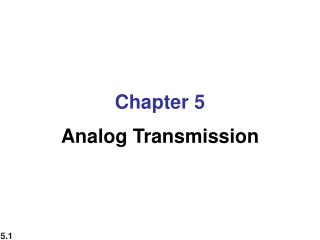# Chapter 5 Analog Transmission - PowerPoint PPT PresentationDownload PresentationChapter 5 Analog Transmission

Chapter 5 Analog Transmission
Download Presentation## Chapter 5 Analog Transmission

- - - - - - - - - - - - - - - - - - - - - - - - - - - E N D - - - - - - - - - - - - - - - - - - - - - - - - - - -
##### Presentation Transcript

1. Chapter 5 Analog Transmission

2. 5-1 DIGITAL-TO-ANALOG CONVERSION Digital-to-analog conversion is the process of changing one of the characteristics of an analog signal based on the information in digital data.

3. Figure 5.1 Digital-to-analog conversion

4. Figure 5.2 Types of digital-to-analog conversion

5. 1. Data element vs. signal element 2. Bit rate is the number of bits per second. 2. Baud rate is the number of signal elements per second. 3. In the analog transmission of digital data, the baud rate is less than or equal to the bit rate. S = N x 1/r baud r = log2L

6. Example 5.1 An analog signal carries 4 bits per signal element. If 1000 signal elements are sent per second, find the bit rate. Solution In this case, r = 4, S = 1000, and N is unknown. We can find the value of N from

7. Example 5.2 An analog signal has a bit rate of 8000 bps and a baud rate of 1000 baud. How many data elements are carried by each signal element? How many signal elements do we need? Solution In this example, S = 1000, N = 8000, and r and L are unknown. We find first the value of r and then the value of L.

8. The amplitude (or height) of the sine wave varies to transmit the ones and zeros Major disadvantage is that telephone lines are very susceptible to variations in transmission quality that can affect amplitude Amplitude Shift Keying (ASK)

9. ASK Illustration 1 0 0 1

10. Figure 5.3 Binary amplitude shift keying B = (1+d) x S = (1+d) x N x 1/r

11. Figure 5.4 Implementation of binary ASK

12. Example 5.3 We have an available bandwidth of 100 kHz which spans from 200 to 300 kHz. What are the carrier frequency and the bit rate if we modulated our data by using ASK with d = 1? Solution The middle of the bandwidth is located at 250 kHz. This means that our carrier frequency can be at fc = 250 kHz. We can use the formula for bandwidth to find the bit rate (with d = 1 and r = 1).

13. Figure 5.6 Binary frequency shift keying

14. Example 5.5 We have an available bandwidth of 100 kHz which spans from 200 to 300 kHz. What should be the carrier frequency and the bit rate if we modulated our data by using FSK with d = 1? Solution This problem is similar to Example 5.3, but we are modulating by using FSK. The midpoint of the band is at 250 kHz. We choose 2Δf to be 50 kHz; this means

15. Figure 5.9 Binary phase shift keying

16. Figure 5.12 Concept of a constellation diagram

17. Figure 5.13 Three constellation diagrams

18. QAM – Quadrature Amplitude Modulation • Modulation technique used in the cable/video networking world • Instead of a single signal change representing only 1 bps – multiple bits can be represented by a single signal change • Combination of phase shifting and amplitude shifting (8 phases, 2 amplitudes)

19. Figure 5.14 Constellation diagrams for some QAMs

20. 5-2 ANALOG-TO-ANALOG CONVERSION Analog-to-analog conversion is the representation of analog information by an analog signal. Modulation is needed if the medium is bandpass in nature or if only a bandpass channel is available to us. Example: radio stations

21. Figure 5.15 Types of analog-to-analog modulation

22. Figure 5.16 Amplitude modulation The total bandwidth required for AM can be determined from the bandwidth of the audio signal: BAM = 2B.

23. Figure 5.17 AM band allocation

24. Figure 5.18 Frequency modulation

25. Figure 5.19 FM band allocation The total bandwidth required for FM can be determined from the bandwidth of the audio signal: BFM = 2(1 + β)B. β has a common value of 4

26. Figure 5.20 Phase modulation The total bandwidth required for PM can be determined from the bandwidth and maximum amplitude of the modulating signal:BPM = 2(1 + β)B.